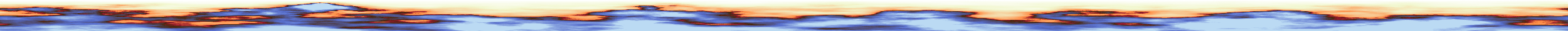# 💲10-SBD Price- ❓Steemit Quiz Contest❓ - Quiz #1 - Here we go again, have fun ✌

in #contest4 years ago# Here we go with the first quiz, have fun!# Quiz #1# The quiz will be resolved the next day.# This is how it works:

### ❗❗You can only comment once and editing your comment is forbidden❗❗# 👉 Upvote this post 👈

(applies for all steemit user! If you want to get the point, you have to upvote this post)

# 👉 Resteem this post 👈

(applies only to user, who did not resteem this contest so far. One resteem is enough!)

# 👉 And of course comment the right answer 👈

Sort:

27 kg

The answer for this quiz is 27.
The cat has a weight of 7.
The rabbit has a weight of 3.
The dog has a weight of 17.
17 + 7 + 3 = 27

it's 27, but you forgot to add "kg"

4 years ago (edited)

Thanks

27kg but it appears I'm late lol

lollll

I posted the answer earlier. This is just additional explanation for anyone interested! :)
a+b = 10
b+c = 20
a+c = 24
a + 20 - b = 24
a - b = 4
a = 4 + b
4 + b + b = 10 -> 2b = 6 -> b = 3
a = 10 - 3 = 7
c = 20 - 3 = 17
a = 7
b = 3
c = 17
a+b+c = 27 kg

good